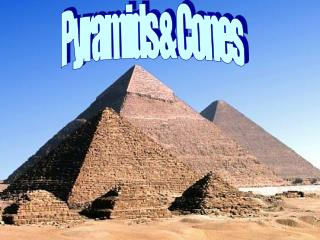DownloadDownload PresentationPyramids & Cones

# Pyramids & Cones

Download Presentation## Pyramids & Cones

- - - - - - - - - - - - - - - - - - - - - - - - - - - E N D - - - - - - - - - - - - - - - - - - - - - - - - - - -
##### Presentation Transcript

1. Pyramids & Cones

2. l 3 8 Practice Pythagorean Theorem a2 + b2 = c2 Find Lateral Area, Surface Area & Volume Surface Area = LA + B 32 + 42 = x2 X = 5 80 + 82 = 144 u2 Lateral Area = ½pl Volume =  B h ½ (32) (5) = 80 u2 (1/3) (64) (3) = 64 u3

3. The Luxor Hotel in Las Vegas, Nevada, is shaped like a gigantic black glass pyramid. The base of the pyramid is a square with edges 606 feet long. The hotel is 350 feet tall. Find the area of the glass on the Luxor. Find the Slant height: L 2 = height2 + (apothem of base)2 L 2 = 3502 + 3032 l = 462.9 Lateral Area = ½ p l LA = ½ (4  606)  462.9 LA = 561,034.8 ft2

4. Name the pyramid. 47 m Pentagonal pyramid Find the lateral surface area. LA = ½(32)(5)(47) 32 m LA = 3760 m2

5. Diameter = 24 Find Lateral Area, Surface Area & Volume x2 = 122 + 82 x = 14.4 8 x Lateral Area = πrl Surface Area = LA + B SA = πrl+ πr2 LA = (3.14) (12) (14.4)LA = 542.6 u2 SA = 542.6 + 3.14 (12)2SA = 994.8 u2 Volume =  B h (1/3) (452.2) (8)= 1205.8 u3

6. The largest tepee in the United States belongs to Dr. Michael Doss of Washington, DC. Dr. Doss is a member of Montana’s Crow Tribe. The tepee is a cone with a diameter of 42 feet and a slant height of 47.9 feet. How much canvas was used to cover the tepee? LA = πrl LA = (π) (21) (47.9) LA = 1005.9 π ft2